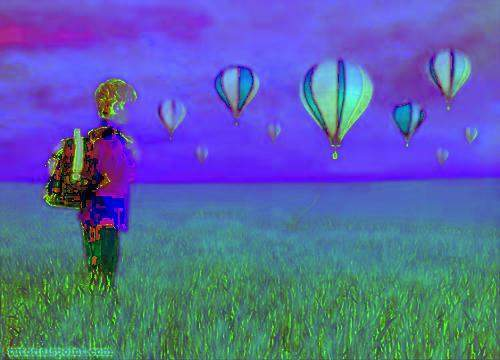# Java DIP - Introduction

Digital Image Processing (DIP) deals with manipulation of digital images using a digital computer. It is a sub field of signals and systems but focuses particularly on images. DIP focuses on developing a computer system that is able to perform processing on an image. The input of such system is a digital image. The system processes the image using efficient algorithms, and gives an image as an output.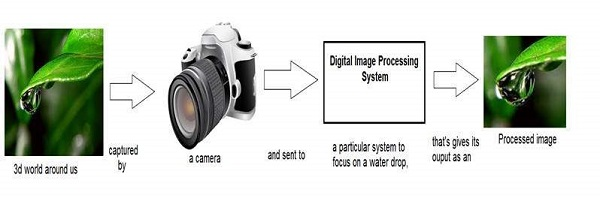Java is a high level programming language that is widely used in the modern world. It can support and handle digital image processing efficiently using various functions.

# Java BufferedImage Class

Java `BufferedImage` class is a subclass of Image class. It is used to handle and manipulate the image data. A `BufferedImage` is made of ColorModel of image data. All `BufferedImage` objects have an upper left corner coordinate of (0, 0).

## Constructors

This class supports three types of constructors.

The first constructor constructs a new `BufferedImage` with a specified ColorModel and Raster.

```BufferedImage(ColorModel cm, WritableRaster raster,
boolean isRasterPremultiplied, Hashtable<?,?> properties)
```

The second constructor constructs a `BufferedImage` of one of the predefined image types.

```BufferedImage(int width, int height, int imageType)
```

The third constructor constructs a `BufferedImage` of one of the predefined image types: TYPE_BYTE_BINARY or TYPE_BYTE_INDEXED.

```BufferedImage(int width, int height, int imageType, IndexColorModel cm)
```

Sr.No Method & Description
1

copyData(WritableRaster outRaster)

It computes an arbitrary rectangular region of the `BufferedImage` and copies it into a specified WritableRaster.

2

getColorModel()

It returns object of class ColorModel of an image.

3

getData()

It returns the image as one large tile.

4

getData(Rectangle rect)

It computes and returns an arbitrary region of the `BufferedImage`.

5

getGraphics()

This method returns a Graphics2D, retains backwards compatibility.

6

getHeight()

It returns the height of the `BufferedImage`.

7

getMinX()

It returns the minimum x coordinate of this `BufferedImage`.

8

getMinY()

It returns the minimum y coordinate of this `BufferedImage`.

9

getRGB(int x, int y)

It returns an integer pixel in the default RGB color model (TYPE_INT_ARGB) and default sRGB colorspace.

10

getType()

It returns the image type.

## Example

The following example demonstrates the use of java `BufferedImage` class that draw some text on the screen using Graphics Object −

```import java.awt.Graphics;
import java.awt.Image;
import java.awt.image.BufferedImage;

import javax.swing.JFrame;
import javax.swing.JPanel;

public class Test extends JPanel {

public void paint(Graphics g) {
Image img = createImageWithText();
g.drawImage(img, 20,20,this);
}

private Image createImageWithText() {
BufferedImage bufferedImage = new BufferedImage(200,200,BufferedImage.TYPE_INT_RGB);
Graphics g = bufferedImage.getGraphics();

g.drawString("www.tutorialspoint.com", 20,20);
g.drawString("www.tutorialspoint.com", 20,40);
g.drawString("www.tutorialspoint.com", 20,60);
g.drawString("www.tutorialspoint.com", 20,80);
g.drawString("www.tutorialspoint.com", 20,100);

return bufferedImage;
}

public static void main(String[] args) {
JFrame frame = new JFrame();

frame.setDefaultCloseOperation(JFrame.EXIT_ON_CLOSE);
frame.setSize(200, 200);
frame.setVisible(true);
}
}
```

## Output

When you execute the given code, the following output is seen −In this chapter we are going to see how you can download an image from internet, perform some image processing techniques on the image, and then again upload the processed image to a server.

In order to download an image from a website, we use java class named URL, which can be found under java.net package. Its syntax is given below −

```String website = "http://tutorialspoint.com";
URL url = new URL(website);
```

Apart from the above method, there are other methods available in class URL as described briefly −

Sr.No. Method & Description
1

public String getPath()

It returns the path of the URL.

2

public String getQuery()

It returns the query part of the URL.

3

public String getAuthority()

It returns the authority of the URL.

4

public int getPort()

It returns the port of the URL.

5

public int getDefaultPort()

It returns the default port for the protocol of the URL.

6

public String getProtocol()

It returns the protocol of the URL.

7

public String getHost()

It returns the host of the URL.

### Example

The following example demonstrates the use of java URL class to download an image from the internet −

```import java.io.FileOutputStream;
import java.io.IOException;
import java.io.InputStream;
import java.io.OutputStream;

import java.net.URL;

public static void main(String[] args) throws Exception {

try{
String fileName = "digital_image_processing.jpg";
String website = "http://tutorialspoint.com/java_dip/images/"+fileName;

URL url = new URL(website);
InputStream inputStream = url.openStream();
OutputStream outputStream = new FileOutputStream(fileName);
byte[] buffer = new byte;

int length = 0;

while ((length = inputStream.read(buffer)) != -1) {
System.out.println("Buffer Read of length: " + length);
outputStream.write(buffer, 0, length);
}

inputStream.close();
outputStream.close();

} catch(Exception e) {
System.out.println("Exception: " + e.getMessage());
}
}
}
```

### Output

When you execute the given above, the following output is seen.Let us see how to upload an image to a webserver. We convert a BufferedImage to byte array in order to send it to server.

We use Java class ByteArrayOutputStream, which can be found under java.io package. Its syntax is given below −

```ByteArrayOutputStream baos = new ByteArrayOutputStream();
ImageIO.write(image, "jpg", baos);
```

In order to convert the image to byte array, we use toByteArray() method of ByteArrayOutputStream class. Its syntax is given below −

```byte[] bytes = baos.toByteArray();
```

Apart from the above method, there are other methods available in the ByteArrayOutputStream class as described briefly −

Sr.No. Method & Description
1

public void reset()

This method resets the number of valid bytes of the byte array output stream to zero, so that all the accumulated output in the stream is discarded.

2

public byte[] toByteArray()

This method creates a newly allocated Byte array. Its size would be the current size of the output stream and the contents of the buffer will be copied into it. It returns the current contents of the output stream as a byte array.

3

public String toString()

Converts the buffer content into a string. Translation will be done according to the default character encoding. It returns the String translated from the buffer's content.

4

public void write(int w)

It writes the specified array to the output stream.

5

public void write(byte []b, int of, int len)

It writes len number of bytes starting from offset off to the stream.

6

public void writeTo(OutputStream outSt)

It writes the entire content of this Stream to the specified stream argument.

### Example

The following example demonstrates ByteArrayOutputStream to upload an image to the server −

#### Client Code

```import javax.swing.*;
import java.net.*;
import java.awt.image.*;
import javax.imageio.*;
import java.io.*;
import java.awt.image.BufferedImage;
import java.io.ByteArrayOutputStream;
import java.io.File;
import java.io.IOException;
import javax.imageio.ImageIO;

public class Client{
public static void main(String args[]) throws Exception{

Socket soc;
BufferedImage img = null;
soc=new Socket("localhost",4000);
System.out.println("Client is running. ");

try {
ByteArrayOutputStream baos = new ByteArrayOutputStream();

ImageIO.write(img, "jpg", baos);
baos.flush();

byte[] bytes = baos.toByteArray();
baos.close();

System.out.println("Sending image to server. ");

OutputStream out = soc.getOutputStream();
DataOutputStream dos = new DataOutputStream(out);

dos.writeInt(bytes.length);
dos.write(bytes, 0, bytes.length);

System.out.println("Image sent to server. ");

dos.close();
out.close();

} catch (Exception e) {
System.out.println("Exception: " + e.getMessage());
soc.close();
}
soc.close();
}
}
```

#### Server Code

```import java.net.*;
import java.io.*;
import java.awt.image.*;

import javax.imageio.*;
import javax.swing.*;

class Server {
public static void main(String  args[]) throws Exception{
ServerSocket server=null;
Socket socket;
server = new ServerSocket(4000);
System.out.println("Server Waiting for image");

socket = server.accept();
System.out.println("Client connected.");

InputStream in = socket.getInputStream();
DataInputStream dis = new DataInputStream(in);

System.out.println("Image Size: " + len/1024 + "KB");

byte[] data = new byte[len];
dis.close();
in.close();

InputStream ian = new ByteArrayInputStream(data);

JFrame f = new JFrame("Server");
ImageIcon icon = new ImageIcon(bImage);
JLabel l = new JLabel();

l.setIcon(icon);
f.pack();
f.setVisible(true);
}
}
```

### Output

#### Client Side Output

When you execute the client code, the following output appears on client side −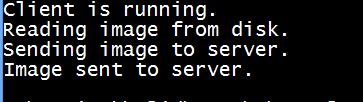#### Server Side Output

When you execute the server code, the following ouptut appears on server side −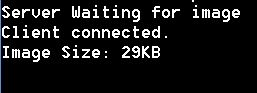After receiving the image, the server displays the image as shown below −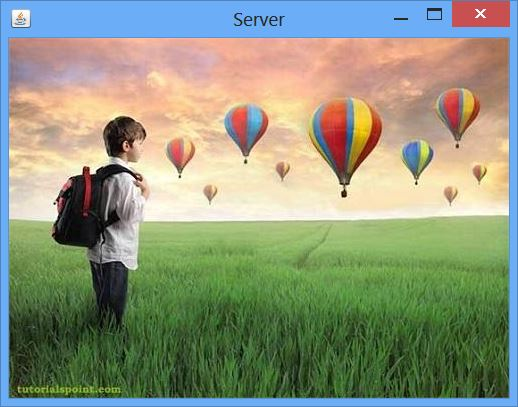# Java DIP - Image Pixels

An image contains a two dimensional array of pixels. It is actually the value of those pixels that make up an image. Usually an image could be color or grayscale.

In Java, the BufferedImage class is used to handle images. You need to call getRGB() method of the BufferedImage class to get the value of the pixel.

## Getting Pixel Value

The pixel value can be received using the following syntax−

```Color c = new Color(image.getRGB(j, i));
```

## Getting RGB Values

The method getRGB() takes the row and column index as a parameter and returns the appropriate pixel. In case of color image, it returns three values which are (Red, Green, Blue). They can be get as follows−

```c.getRed();
c.getGreen();
c.getBlue();
```

## Getting Width and Height of Image

The height and width of the image can be get by calling the getWidth() and getHeight() methods of the BufferedImage class. Its syntax is given below−

```int width = image.getWidth();
int height = image.getHeight();
```

Apart from these methods, there are other methods supported in the BufferedImage class. They are described briefly−

Sr.No. Method & Description
1

copyData(WritableRaster outRaster)

It computes an arbitrary rectangular region of the BufferedImage and copies it into a specified WritableRaster.

2

getColorModel()

It returns ColorModel of an image.

3

getData()

It returns the image as one large tile.

4

getData(Rectangle rect)

It computes and returns an arbitrary region of the BufferedImage.

5

getGraphics()

This method returns a Graphics2D, but is here for backwards compatibility.

6

getHeight()

It returns the height of the BufferedImage.

7

getMinX()

It returns the minimum x coordinate of this BufferedImage.

8

getMinY()

It returns the minimum y coordinate of this BufferedImage.

9

getRGB(int x, int y)

It returns an integer pixel in the default RGB color model (TYPE_INT_ARGB) and default sRGB colorspace.

10

getType()

It returns the image type.

### Example

The following example demonstrates the use of java BufferedImage class that displays pixels of an image of size (100 x 100)−

```import java.awt.*;
import java.awt.image.BufferedImage;

import java.io.*;

import javax.imageio.ImageIO;
import javax.swing.JFrame;

class Pixel {
BufferedImage image;
int width;
int height;

public Pixel() {
try {
File input = new File("blackandwhite.jpg");
width = image.getWidth();
height = image.getHeight();

int count = 0;

for(int i=0; i<height; i++) {

for(int j=0; j<width; j++) {

count++;
Color c = new Color(image.getRGB(j, i));
System.out.println("S.No: " + count + " Red: " + c.getRed() +"  Green: " + c.getGreen() + " Blue: " + c.getBlue());
}
}

} catch (Exception e) {}
}

static public void main(String args[]) throws Exception {
Pixel obj = new Pixel();
}
}
```

### Output

When you execute the above example, it would print the pixels of the following image −

Original ImagePixels Output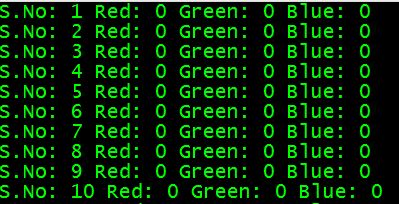If you scroll down the ouput, the following pattern is seen−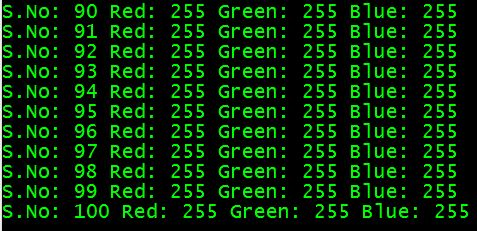# Java DIP - GrayScale Conversion

In order to convert a color image to Grayscale image, you need to read pixels or data of the image using File and ImageIO objects, and store the image in BufferedImage object. Its syntax is given below −

```File input = new File("digital_image_processing.jpg");
```

Further, get the pixel value using method getRGB() and perform GrayScale() method on it. The method getRGB() takes row and column index as parameter.

```Color c = new Color(image.getRGB(j, i));
int red = (c.getRed() * 0.299);
int green =(c.getGreen() * 0.587);
int blue = (c.getBlue() *0.114);
```

Apart from these three methods, there are other methods available in the Color class as described briefly −

Sr.No. Method & Description
1

brighter()

It creates a new Color that is a brighter version of this Color.

2

darker()

It creates a new Color that is a darker version of this Color.

3

getAlpha()

It returns the alpha component in the range 0-255.

4

getHSBColor(float h, float s, float b)

It creates a Color object based on the specified values for the HSB color model.

5

HSBtoRGB(float hue, float saturation, float brightness)

It converts the components of a color, as specified by the HSB model, to an equivalent set of values for the default RGB model.

6

toString()

It returns a string representation of this Color.

The last step is to add all these three values and set it again to the corresponding pixel value. Its syntax is given below −

```int sum = red+green+blue;
Color newColor = new Color(sum,sum,sum);
image.setRGB(j,i,newColor.getRGB());
```

## Example

The following example demonstrates the use of Java BufferedImage class that converts an image to Grayscale −

```import java.awt.*;
import java.awt.image.BufferedImage;

import java.io.*;

import javax.imageio.ImageIO;
import javax.swing.JFrame;

public class GrayScale {

BufferedImage  image;
int width;
int height;

public GrayScale() {

try {
File input = new File("digital_image_processing.jpg");
width = image.getWidth();
height = image.getHeight();

for(int i=0; i<height; i++) {

for(int j=0; j<width; j++) {

Color c = new Color(image.getRGB(j, i));
int red = (int)(c.getRed() * 0.299);
int green = (int)(c.getGreen() * 0.587);
int blue = (int)(c.getBlue() *0.114);
Color newColor = new Color(red+green+blue,

red+green+blue,red+green+blue);

image.setRGB(j,i,newColor.getRGB());
}
}

File ouptut = new File("grayscale.jpg");
ImageIO.write(image, "jpg", ouptut);

} catch (Exception e) {}
}

static public void main(String args[]) throws Exception {
GrayScale obj = new GrayScale();
}
}
```

## Output

When you execute the given example, it converts the image digital_image_processing.jpg to its equivalent Grayscale image and write it on hard disk with the name grayscale.jpg.

### Original Image### Grayscale Image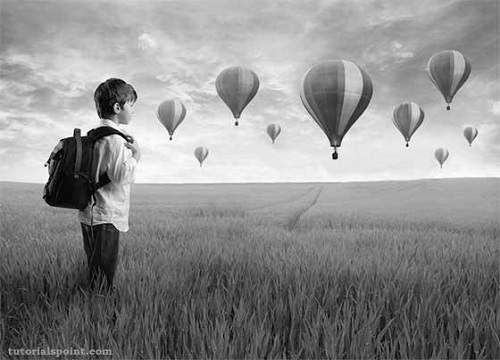# Java DIP - Enhancing Image Contrast

In this chapter learn how to enhance the contrast of an image using histogram equalization.

We use the OpenCV function equalizeHist() method. It can be found under Imgproc package. Its syntax is given below −

```Imgproc.equalizeHist(source, destination);
```

The parameters are described below −

Sr.No. Parameter & Description
1

Source

It is 8-bit single channel source image.

2

Destination

It is the destination image.

Apart from the equalizeHist() method, there are other methods provided by the Imgproc class. They are described briefly −

Sr.No. Method & Description
1

cvtColor(Mat src, Mat dst, int code, int dstCn)

It converts an image from one color space to another.

2

dilate(Mat src, Mat dst, Mat kernel)

It dilates an image by using a specific structuring element.

3

equalizeHist(Mat src, Mat dst)

It equalizes the histogram of a grayscale image.

4

filter2D(Mat src, Mat dst, int ddepth, Mat kernel, Point anchor, double delta)

It convolves an image with the kernel.

5

GaussianBlur(Mat src, Mat dst, Size ksize, double sigmaX)

It blurs an image using a Gaussian filter.

6

integral(Mat src, Mat sum)

It calculates the integral of an image.

## Example

The following example demonstrates the use of Imgproc class to enhance contrast of an image −

```import org.opencv.core.Core;
import org.opencv.core.Mat;
import org.opencv.highgui.Highgui;
import org.opencv.imgproc.Imgproc;

public class Main {

static int width;
static int height;
static double alpha = 2;
static double beta = 50;

public static void main( String[] args ) {

try {
Mat destination = new Mat(source.rows(),source.cols(),source.type());

Imgproc.equalizeHist(source, destination);
Highgui.imwrite("contrast.jpg", destination);

} catch (Exception e) {
System.out.println("error: " + e.getMessage());
}
}
}
```

## Output

When you execute the given code, the following output is seen −

### Original Image### Enhanced Contrast Image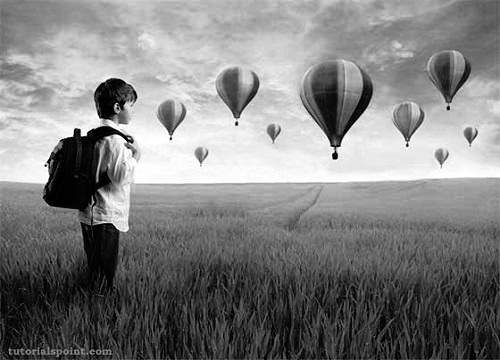# Java DIP - Enhancing Image Brightness

In this chapter we enhance the brightness of an image by multiplying each pixel of the image with an alpha value and adding another beta value to it.

We OpenCV function convertTo that does the above operation automatically. It can be found under Mat package. Its syntax is given below −

```int alpha = 2;
int beta = 50;
sourceImage.convertTo(destination, rtype , alpha, beta);
```

The parameters are described below −

Sr.No. Parameter & Description
1

destination

It is destination image.

2

rtype

It is desired output matrix type or, rather the depth, since the number of channels are the same as the input has. if rtype is negative, the output matrix will have the same type as the input.

3

alpha

It is optional scale factor.

4

beta

It is optional delta added to the scaled values.

Apart from the convertTo method, there are other methods provided by the Mat class. They are described briefly −

Sr.No. Method & Description
1

adjustROI(int dtop, int dbottom, int dleft, int dright)

It adjusts a submatrix size and position within the parent matrix.

2

copyTo(Mat m)

It copies the matrix to another one.

3

diag()

It extracts a diagonal from a matrix, or creates a diagonal matrix.

4

dot(Mat m)

It computes a dot-product of two vectors.

5

reshape(int cn)

It changes the shape and/or the number of channels of a 2D matrix without copying the data.

6

submat(Range rowRange, Range colRange)

It extracts a rectangular sub matrix.

## Example

The following example demonstrates the use of Mat class to enhance brightness of an image −

```import org.opencv.core.Core;
import org.opencv.core.Mat;
import org.opencv.highgui.Highgui;

public class Main {
static int width;
static int height;
static double alpha = 2;
static double beta = 50;

public static void main( String[] args ) {

try{
Mat destination = new Mat(source.rows(),source.cols(),

source.type());
source.convertTo(destination, -1, alpha, beta);
Highgui.imwrite("brightWithAlpha2Beta50.jpg", destination);

} catch (Exception e) {
System.out.println("error:" + e.getMessage());
}
}
}
```

## Output

When you execute the given code, the following output is seen −

### Original Image### Enhanced Bright Image (Alpha=1 & Beta=50)### Enhanced Bright Image (Alpha=2 & Beta=50)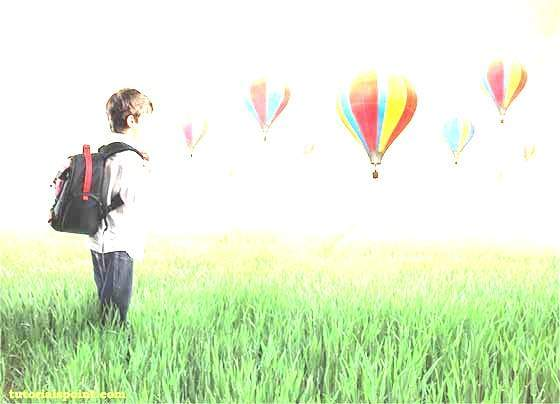# Java DIP - Enhancing Image Sharpness

In this chapter we learn to increase the sharpness of an image using Gaussian filter.

First we use OpenCV function GaussianBlur. It can be found under Imgproc package. Its syntax is given below −

```Imgproc.GaussianBlur(source, destination, new Size(0,0), sigmaX);
```

The parameters are described briefly −

Sr.No. Parameter & Description
1

source

It is source image.

2

destination

It is destination image.

3

Size

It is Gaussian kernel size.

4

sigmaX

It is Gaussian kernel standard deviation in X direction.

Further, we use OpenCV function addWeighted to apply image watermark to image. It can be found under Core package. Its syntax is given below −

```Core.addWeighted(InputArray src1, alpha, src2, beta, gamma, OutputArray dst);
```

The parameters of this function are described below −

Sr.No. Parameter & Description
1

src1

It is first input array.

2

alpha

It is weight of the first array elements.

3

src2

It is second input array of the same size and channel number as src1.

4

Beta

It is weight of the second array elements.

5

gamma

It is scalar added to each sum.

6

dst

It is output array that has the same size and number of channels as the input arrays.

Apart from the GaussianBlur method, there are other methods provided by the Imgproc class. They are described briefly −

Sr.No. Method & Description
1

cvtColor(Mat src, Mat dst, int code, int dstCn)

It converts an image from one color space to another.

2

dilate(Mat src, Mat dst, Mat kernel)

It dilates an image by using a specific structuring element.

3

equalizeHist(Mat src, Mat dst)

It equalizes the histogram of a grayscale image.

4

filter2D(Mat src, Mat dst, int depth, Mat kernel, Point anchor, double delta)

It convolves an image with the kernel.

5

GaussianBlur(Mat src, Mat dst, Size ksize, double sigmaX)

It blurs an image using a Gaussian filter.

6

integral(Mat src, Mat sum)

It calculates the integral of an image.

## Example

The following example demonstrates the use of Imgproc and Core class to apply sharpening to an image −

```import org.opencv.core.Core;
import org.opencv.core.Mat;
import org.opencv.core.Size;
import org.opencv.highgui.Highgui;
import org.opencv.imgproc.Imgproc;

public class Main {
public static void main( String[] args ) {
try{
Mat destination = new Mat(source.rows(),source.cols(),source.type());
Imgproc.GaussianBlur(source, destination, new Size(0,0), 10);
Core.addWeighted(source, 1.5, destination, -0.5, 0, destination);
Highgui.imwrite("sharp.jpg", destination);
} catch (Exception e) {
}
}
}
```

## Output

When you execute the given code, the following output is seen −

### Original Image### Sharped Image# Java DIP - Image Compression Technique

An image can easily be compressed and stored through Java. Compression of image involves converting an image into jpg and storing it.

In order to compress an image, we read the image and convert into BufferedImage object.

Further, we get an ImageWriter from getImageWritersByFormatName() method found in the ImageIO class. From this ImageWriter, create an ImageWriteParam object. Its syntax is given below −

```Iterator<ImageWriter> list = ImageIO.getImageWritersByFormatName("jpg");
ImageWriteParam obj = writer_From_List.getDefaultWriteParam();
```

From this ImageWriteParam object, you can set the compression by calling these two methods which are setCompressionMode() and setCompressionQuality(). Their syntaxes are as given below −

```obj.setCompressionMode(ImageWriteParam.MODE_EXPLICIT);
obj.setCompressionQuality(0.05f);
```

The setCompressionMode() method takes Mode_EXPLICIT as the parameter. Some of the other MODES are described briefly −

Sr.No. Modes
1

MODE_DEFAULT

It is a constant value that may be passed into methods to enable that feature for future writes.

2

MODE_DISABLED

It is a constant value that may be passed into methods to disable that feature for future writes.

3

MODE_EXPLICIT

It is a constant value that may be passed into methods to enable that feature for future writes.

Apart from the compressions methods, there are other methods provided by the ImageWriteParam class. They are described briefly −

Sr.No. Method & Description
1

canOffsetTiles()

It returns true if the writer can perform tiling with non-zero grid offsets while writing.

2

getBitRate(float quality)

It returns a float indicating an estimate of the number of bits of output data for each bit of input image data at the given quality level.

3

getLocale()

It returns the currently set Locale, or null if only a default Locale is supported.

4

isCompressionLossless()

It returns true if the current compression type provides lossless compression.

5

unsetCompression()

It removes any previous compression type and quality settings.

6

unsetTiling()

It removes any previous tile grid parameters specified by calls to setTiling.

## Example

The following example demonstrates the use of ImageWriteParam class to compress an image −

```import java.io.*;
import java.util.*;
import java.awt.image.*;

import javax.imageio.*;
import javax.imageio.stream.ImageOutputStream;

class Compression {

public static void main(String[] args) throws IOException {

File input = new File("digital_image_processing.jpg");

File compressedImageFile = new File("compress.jpg");
OutputStream os =new FileOutputStream(compressedImageFile);

Iterator<ImageWriter>writers =  ImageIO.getImageWritersByFormatName("jpg");
ImageWriter writer = (ImageWriter) writers.next();

ImageOutputStream ios = ImageIO.createImageOutputStream(os);
writer.setOutput(ios);

ImageWriteParam param = writer.getDefaultWriteParam();

param.setCompressionMode(ImageWriteParam.MODE_EXPLICIT);
param.setCompressionQuality(0.05f);
writer.write(null, new IIOImage(image, null, null), param);

os.close();
ios.close();
writer.dispose();
}
}
```

## Output

When you execute the given code, it compresses the image digital_image_processing.jpg to its equivalent compressed image and writes it on the hard disk with the name compress.jpg.

### Original Image### Compressed Image - Quality Factor − 0.05### Compressed Image - Quality Factor − 0.5# Java DIP - Adding Border

In this chapter we learn to add different types of borders to an image.

We use OpenCV function copyMakeBorder. It can be found under Imgproc package. Its syntax is given below −

```Imgproc.copyMakeBorder(source,destination,top,bottom,left,right,borderType);
```

The parameters are described below −

Sr.No. Parameter & Description
1

source

It is source image.

2

destination

It is destination image.

3

top

It is the length in pixels of the border at the top of the image.

4

bottom

Length in pixels of the border at the bottom of the image.

5

left

It is the length in pixels of the border at the left of the image.

6

right

It is the length in pixels of the border at the right of the image.

7

borderType

It defines the type of border. The possible borders are BORDER_REPLICATE, BORDER_REFLECT, BORDER_WRAP, BORDER_CONSTANT etc.

Apart from the copyMakeBorder() method, there are other methods provide by the Imgproc class. They are described briefly −

Sr.No. Method & Description
1

cvtColor(Mat src, Mat dst, int code, int dstCn)

It converts an image from one color space to another.

2

dilate(Mat src, Mat dst, Mat kernel)

It dilates an image by using a specific structuring element.

3

equalizeHist(Mat src, Mat dst)

It equalizes the histogram of a grayscale image.

4

filter2D(Mat src, Mat dst, int depth, Mat kernel, Point anchor, double delta)

It convolves an image with the kernel.

5

GaussianBlur(Mat src, Mat dst, Size ksize, double sigmaX)

It blurs an image using a Gaussian filter.

6

integral(Mat src, Mat sum)

It calculates the integral of an image.

## Example

The following example demonstrates the use of Imgproc class to add border to an image −

```import org.opencv.core.Core;
import org.opencv.core.CvType;
import org.opencv.core.Mat;

import org.opencv.highgui.Highgui;
import org.opencv.imgproc.Imgproc;

public class main {
public static void main( String[] args ) {

try {

Mat destination = new Mat(source.rows(),source.cols(),source.type());

int top, bottom, left, right;
int borderType;

/// Initialize arguments for the filter
top = (int) (0.05*source.rows());
bottom = (int) (0.05*source.rows());
left = (int) (0.05*source.cols());
right = (int) (0.05*source.cols());

destination = source;
Imgproc.copyMakeBorder(source, destination, top, bottom, left, right, Imgproc.BORDER_WRAP);
Highgui.imwrite("borderWrap.jpg", destination);

} catch (Exception e) {
System.out.println("error: " + e.getMessage());
}
}
}
```

## Output

When you execute the given code, the following output is seen −

### Original Image### Isolated Border Image### Wrapped Border Image### Reflect Border Image# Java DIP - Image Pyramids

Image pyramid is nothing but a method to display a multi-resolution image. The lowermost layer is a highest-resolution version of image and the topmost layer is a lowest-resolution version of the image. Image pyramids are used to handle image at different scales.

In this chapter we perform some down sampling and up sampling on images.

We use OpenCV functions pyrUp and pyrDown. They can be found under Imgproc package. Its syntax is given below −

```Imgproc.pyrUp(source, destination, destinationSize);
Imgproc.pyrDown(source, destination,destinationSize);
```

The parameters are described below −

Sr.No. Parameter & Description
1

source

It is the source image.

2

destination

It is the destination image.

3

destinationSize

It is the size of the output image. By default, it is computed as Size((src.cols*2), (src.rows*2)).

Apart from the pyrUp and pyrDown methods, there are other methods provided by the Imgproc class. They are described briefly −

Sr.No. Method & Description
1

cvtColor(Mat src, Mat dst, int code, int dstCn)

It converts an image from one color space to another.

2

dilate(Mat src, Mat dst, Mat kernel)

It dilates an image by using a specific structuring element.

3

equalizeHist(Mat src, Mat dst)

It equalizes the histogram of a grayscale image.

4

filter2D(Mat src, Mat dst, int depth, Mat kernel, Point anchor, double delta)

It convolves an image with the kernel.

5

GaussianBlur(Mat src, Mat dst, Size ksize, double sigmaX)

It blurs an image using a Gaussian filter.

6

integral(Mat src, Mat sum)

It calculates the integral of an image.

## Example

The following example demonstrates the use of Imgproc class to perform up sampling and down sampling on an image.

```import org.opencv.core.Core;
import org.opencv.core.CvType;
import org.opencv.core.Mat;
import org.opencv.core.Size;

import org.opencv.highgui.Highgui;
import org.opencv.imgproc.Imgproc;

public class main {
public static void main( String[] args ) {

try{

Mat destination1 = new Mat(source.rows()*2, source.cols()*2,source.type());
destination1 = source;

Imgproc.pyrUp(source, destination1, new  Size(source.cols()*2   source.rows()*2));
Highgui.imwrite("pyrUp.jpg", destination1);

Mat destination = new Mat(source.rows()/2,source.cols()/2, source.type());
destination = source;
Imgproc.pyrDown(source, destination, new Size(source.cols()/2,  source.rows()/2));
Highgui.imwrite("pyrDown.jpg", destination);

} catch (Exception e) {
System.out.println("error: " + e.getMessage());
}
}
}
```

## Output

When you execute the given code, the following output is seen −

### Original ImageOn the original image, pyrUp(UP Sampling) and pyrDown(Down Sampling) are performed. The output after sampling is as shown below −

### PyrUP Image### pyrDown Image# Java DIP - Basic Thresholding

Thresholding enables to achieve image segmentation in the easiest way. Image segmentation means dividing the complete image into a set of pixels in such a way that the pixels in each set have some common characteristics. Image segmentation is highly useful in defining objects and their boundaries.

In this chapter we perform some basic thresholding operations on images.

We use OpenCV function threshold. It can be found under Imgproc package. Its syntax is given below −

```Imgproc.threshold(source, destination, thresh , maxval , type);
```

The parameters are described below −

Sr.No. Parameter & Description
1

source

It is source image.

2

destination

It is destination image.

3

thresh

It is threshold value.

4

maxval

It is the maximum value to be used with the THRESH_BINARY and THRESH_BINARY_INV threshold types.

5

type

The possible types are THRESH_BINARY, THRESH_BINARY_INV, THRESH_TRUNC, and THRESH_TOZERO.

Apart from these thresholding methods, there are other methods provided by the Imgproc class. They are described briefly −

Sr.No. Method & Description
1

cvtColor(Mat src, Mat dst, int code, int dstCn)

It converts an image from one color space to another.

2

dilate(Mat src, Mat dst, Mat kernel)

It dilates an image by using a specific structuring element.

3

equalizeHist(Mat src, Mat dst)

It equalizes the histogram of a grayscale image.

4

filter2D(Mat src, Mat dst, int ddepth, Mat kernel, Point anchor, double delta)

It convolves an image with the kernel.

5

GaussianBlur(Mat src, Mat dst, Size ksize, double sigmaX)

It blurs an image using a Gaussian filter.

6

integral(Mat src, Mat sum)

It calculates the integral of an image.

## Example

The following example demonstrates the use of Imgproc class to perform thresholding operations to an image −

```import org.opencv.core.Core;
import org.opencv.core.CvType;
import org.opencv.core.Mat;

import org.opencv.highgui.Highgui;
import org.opencv.imgproc.Imgproc;

public class main {
public static void main( String[] args ) {

try{

Mat destination = new Mat(source.rows(),source.cols(),source.type());

destination = source;
Imgproc.threshold(source,destination,127,255,Imgproc.THRESH_TOZERO);
Highgui.imwrite("ThreshZero.jpg", destination);

} catch (Exception e) {
System.out.println("error: " + e.getMessage());
}
}
}
```

## Output

When you execute the given code, the following output is seen −

### Original ImageOn the above original image, some thresholding operations is performed which is shown in the output below −

### Thresh Binary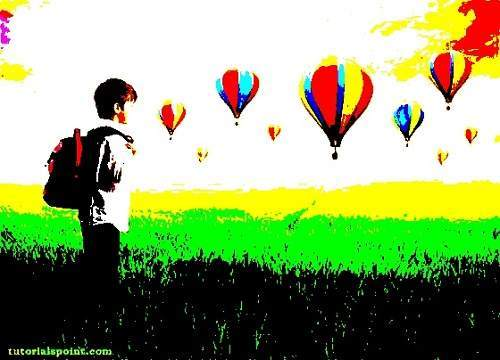### Thresh Binary Invert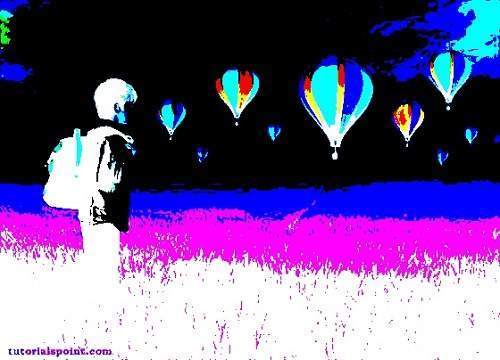### Thresh Zero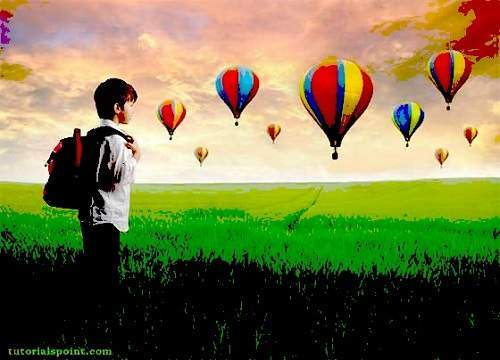# Java DIP - Image Shape Conversion

The shape of the image can easily be changed by using OpenCV. Image can either be flipped, scaled, or rotated in any of the four directions.

In order to change the shape of the image, we read the image and convert into Mat object. Its syntax is given below −

```File input = new File("digital_image_processing.jpg");
//convert Buffered Image to Mat.
```

## Flipping an Image

OpenCV allows three types of flip codes which are described below −

Sr.No. Flip Code & Description
1

0

0 means, flipping around x axis.

2

1

1 means, flipping around y axis.

3

-1

-1 means, flipping around both axis.

We pass the appropriate flip code into method flip() in the Core class. Its syntax is given below −

```Core.flip(source mat, destination mat1, flip_code);
```

The method flip() takes three parameters − the source image matrix, the destination image matrix, and the flip code.

Apart from the flip method, there are other methods provided by the Core class. They are described briefly −

Sr.No. Method & Description
1

add(Mat src1, Mat src2, Mat dst)

It calculates the per-element sum of two arrays or an array and a scalar.

2

bitwise_and(Mat src1, Mat src2, Mat dst)

It calculates the per-element bit-wise conjunction of two arrays or an array and a scalar.

3

bitwise_not(Mat src, Mat dst)

It inverts every bit of an array.

4

circle(Mat img, Point center, int radius, Scalar color)

It draws a circle.

5

sumElems(Mat src)

It blurs an image using a Gaussian filter.

6

subtract(Mat src1, Scalar src2, Mat dst, Mat mask)

It calculates the per-element difference between two arrays or array and a scalar.

### Example

The following example demonstrates the use of Core class to flip an image −

```import java.awt.image.BufferedImage;
import java.awt.image.DataBufferByte;

import java.io.File;
import javax.imageio.ImageIO;

import org.opencv.core.Core;
import org.opencv.core.CvType;
import org.opencv.core.Mat;

import org.opencv.imgproc.Imgproc;

public class Main {
public static void main( String[] args ) {

try {
File input = new File("digital_image_processing.jpg");

byte[] data = ((DataBufferByte) image.getRaster().  getDataBuffer()).getData();
Mat mat = new Mat(image.getHeight(),image.getWidth(),CvType.CV_8UC3);
mat.put(0, 0, data);

Mat mat1 = new Mat(image.getHeight(),image.getWidth(),CvType.CV_8UC3);
Core.flip(mat, mat1, -1);

byte[] data1 = new byte[mat1.rows()*mat1.cols()*(int)(mat1.elemSize())];
mat1.get(0, 0, data1);
BufferedImage image1 = new BufferedImage(mat1.cols(), mat1.rows(), 5);
image1.getRaster().setDataElements(0,0,mat1.cols(),mat1.rows(),data1);

File ouptut = new File("hsv.jpg");
ImageIO.write(image1, "jpg", ouptut);

} catch (Exception e) {
System.out.println("Error: " + e.getMessage());
}
}
}
```

### Output

When you run the above example, it would flip an image name digital_image_processing.jpg to its equivalent HSV color space image and write it on hard disk with name flip.jpg.

#### Original Image#### Flipped Image# Java DIP - Applying Gaussian Filter

In this chapter, we apply Gaussian filter to an image that blurs an image. We are going to use OpenCV function GaussianBlur to apply Gaussian filter to images. It can be found under Imgproc package. Its syntax is given below −

```Imgproc.GaussianBlur(source, destination,Size,SigmaX);
```

The function arguments are described below −

Sr.No. Argument & Description
1

source

It is source image.

2

destination

It is destination image.

3

Size

It is Gaussian kernel size.

4

SigmaX

It is Gaussian kernel standard deviation in X direction.

Apart from the GaussianBlur method, there are other methods provided by the Imgproc class. They are described briefly −

Sr.No. Method & Description
1

cvtColor(Mat src, Mat dst, int code, int dstCn)

It converts an image from one color space to another.

2

dilate(Mat src, Mat dst, Mat kernel)

It dilates an image by using a specific structuring element.

3

equalizeHist(Mat src, Mat dst)

It equalizes the histogram of a grayscale image.

4

filter2D(Mat src, Mat dst, int depth, Mat kernel, Point anchor, double delta)

It convolves an image with the kernel.

5

GaussianBlur(Mat src, Mat dst, Size ksize, double sigmaX)

It blurs an image using a Gaussian filter.

6

integral(Mat src, Mat sum)

It calculates the integral of an image.

## Example

The following example demonstrates the use of Imgproc class to apply Gaussian filter to an image.

```import org.opencv.core.Core;
import org.opencv.core.CvType;
import org.opencv.core.Mat;
import org.opencv.core.Size;

import org.opencv.highgui.Highgui;
import org.opencv.imgproc.Imgproc;

public class Main {
public static void main( String[] args ) {

try {

Mat destination = new Mat(source.rows(),source.cols(),source.type());
Imgproc.GaussianBlur(source, destination,new Size(45,45), 0);
Highgui.imwrite("Gaussian45.jpg", destination);

} catch (Exception e) {
System.out.println("Error:" + e.getMessage());
}
}
}
```

## Output

When you execute the given code, the following output is seen −

### Original ImageWhen this original image is convolved with the Gaussian filter of size 11 and 45, the following output is seen.

### Gaussian filter of size 11### Gaussian filter of size 45# Java DIP - Applying Box Filter

We apply Box filter that blurs an image. A Box filter could be of dimensions 3x3, 5x5, 9x9 etc.

We use OpenCV function filter2D to apply Box filter to images. It can be found under Imgproc package. Its syntax is given below −

```filter2D(src, dst, depth , kernel, anchor, delta, BORDER_DEFAULT );
```

The function arguments are described below −

Sr.No. Argument & Description
1

src

It is source image.

2

dst

It is destination image.

3

depth

It is the depth of dst. A negative value (such as -1) indicates that the depth is the same as the source.

4

kernel

It is the kernel to be scanned through the image.

5

anchor

It is the position of the anchor relative to its kernel. The location Point (-1, -1) indicates the center by default.

6

delta

It is a value to be added to each pixel during the convolution. By default it is 0.

7

BORDER_DEFAULT

We let this value by default.

Apart from the filter2D() method, there are other methods provided by the Imgproc class. They are described briefly −

Sr.No. Method & Description
1

cvtColor(Mat src, Mat dst, int code, int dstCn)

It converts an image from one color space to another.

2

dilate(Mat src, Mat dst, Mat kernel)

It dilates an image by using a specific structuring element.

3

equalizeHist(Mat src, Mat dst)

It equalizes the histogram of a grayscale image.

4

filter2D(Mat src, Mat dst, int depth, Mat kernel, Point anchor, double delta)

It convolves an image with the kernel.

5

GaussianBlur(Mat src, Mat dst, Size ksize, double sigmaX)

It blurs an image using a Gaussian filter.

6

integral(Mat src, Mat sum)

It calculates the integral of an image.

## Example

The following example demonstrates the use of Imgproc class to apply Box filter to an image of Grayscale.

```import org.opencv.core.Core;
import org.opencv.core.CvType;
import org.opencv.core.Mat;

import org.opencv.highgui.Highgui;
import org.opencv.imgproc.Imgproc;

public class convolution {
public static void main( String[] args ) {

try {
int kernelSize = 9;

Mat destination = new Mat(source.rows(),source.cols(),source.type());
Mat kernel = Mat.ones(kernelSize,kernelSize, CvType.CV_32F);

for(int i=0; i<kernel.rows(); i++) {
for(int j=0; j<kernel.cols(); j++) {

double[] m = kernel.get(i, j);

for(int k =0; k<m.length; k++) {
m[k] = m[k]/(kernelSize * kernelSize);
}
kernel.put(i,j, m);
}
}

Imgproc.filter2D(source, destination, -1, kernel);
Highgui.imwrite("output.jpg", destination);

} catch (Exception e) {
System.out.println("Error: " + e.getMessage());
}
}
}
```

## Output

When you execute the given code, the following output is seen −

### Original ImageIn this example we convolve our image with the following filter (kernel). This filter results in blurring an image as its size increases.

This original image has been convolved with the box filter of size 5, which is given below −

### Box filter of size 5

 1/25 1/25 1/25 1/25 1/25 1/25 1/25 1/25 1/25 1/25 1/25 1/25 1/25 1/25 1/25 1/25 1/25 1/25 1/25 1/25 1/25 1/25 1/25 1/25 1/25

### Convolved Image (with Box Filter of Size 5)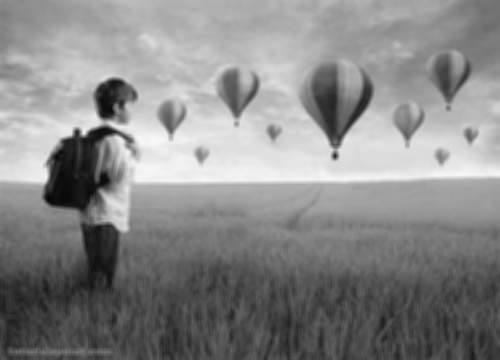### Convolved Image (with Box Filter of Size 9)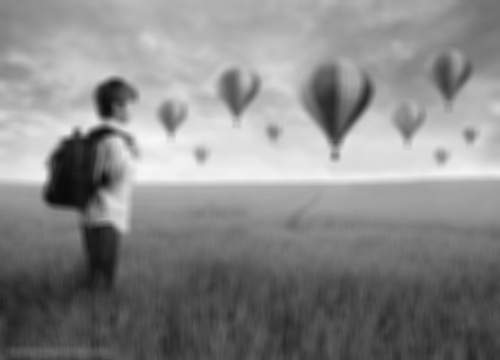# Java DIP - Eroding and Dilating

In this chapter we learn apply two very common morphology operators:Dilation and Erosion.

We use OpenCV function erode and dilate. They can be found under Imgproc package. Its syntax is given below −

```Imgproc.erode(source, destination, element);
Imgproc.dilate(source, destination, element);
```

The parameters are described below −

Sr.No. Parameter & Description
1

source

It is Source image.

2

destination

It is destination image.

3

element

It is a structuring element used for erosion and dilation, if element=Mat(), a 3 x 3 rectangular structuring element is used.

Apart from erode() and dilate() methods, there are other methods provided by the Imgproc class. They are described briefly −

Sr.No. Method & Description
1

cvtColor(Mat src, Mat dst, int code, int dstCn)

It converts an image from one color space to another.

2

dilate(Mat src, Mat dst, Mat kernel)

It dilates an image by using a specific structuring element.

3

equalizeHist(Mat src, Mat dst)

It equalizes the histogram of a grayscale image.

4

filter2D(Mat src, Mat dst, int depth, Mat kernel, Point anchor, double delta)

It convolves an image with the kernel.

5

GaussianBlur(Mat src, Mat dst, Size ksize, double sigmaX)

It blurs an image using a Gaussian filter.

6

integral(Mat src, Mat sum)

It calculates the integral of an image.

## Example

The following example demonstrates the use of Imgproc class to perform erosion and dilation on an image −

```import org.opencv.core.Core;
import org.opencv.core.CvType;
import org.opencv.core.Mat;
import org.opencv.highgui.Highgui;
import org.opencv.imgproc.Imgproc;

public class main {
public static void main( String[] args ) {

try{
Mat destination = new Mat(source.rows(),source.cols(),source.type());

destination = source;

int erosion_size = 5;
int dilation_size = 5;

Mat element = Imgproc.getStructuringElement(Imgproc.MORPH_RECT, new  Size(2*erosion_size + 1, 2*erosion_size+1));
Imgproc.erode(source, destination, element);
Highgui.imwrite("erosion.jpg", destination);

destination = source;

Mat element1 = Imgproc.getStructuringElement(Imgproc.MORPH_RECT, new  Size(2*dilation_size + 1, 2*dilation_size+1));
Imgproc.dilate(source, destination, element1);
Highgui.imwrite("dilation.jpg", destination);

} catch (Exception e) {
System.out.println("error:" + e.getMessage());
}
}
}
```

## Output

When you execute the given code, the following output is seen −

### Original ImageOn the above original image, some erosion and dilation operations have been performed which have been shown in the output below −

### Erosion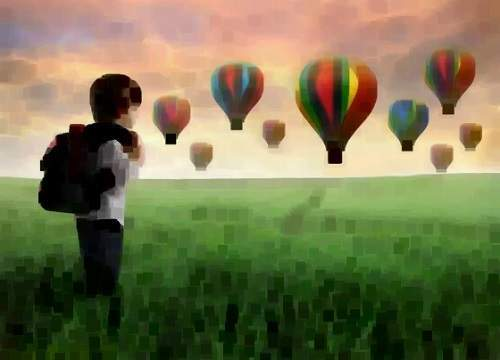### Dilation# Java DIP - Applying Watermark

In this chapter we learn two ways of applying watermark on images. These ways are −

• Applying Text Watermark
• Applying Image watermark

## Applying Text Watermark

We use OpenCV function putText to apply text watermark to image. It can be found under Core package. Its syntax is given below −

```Core.putText(source, Text, Point, fontFace ,fontScale , color);
```

The parameters of this function are described below −

Sr.No. Parameter & Description
1

Source

It is source image.

2

Text

It is the string text that would appear on the image.

3

Point

It is the point where text should appear on image.

4

fontFace

Font type. For example − FONT_HERSHEY_SIMPLEX, FONT_HERSHEY_PLAIN, FONT_HERSHEY_COMPLEX etc.

5

fontScale

It is font scale factor that is multiplied by the font-specific base size.

6

color

It is text color.

Apart from the putText method, there are other methods provided by the Core class. They are described briefly −

Sr.No. Method & Description
1

normalize(Mat src, Mat dst, double alpha, double beta, int norm_type)

It normalizes the norm or value range of an array.

2

perspectiveTransform(Mat src, Mat dst, Mat m)

It performs the perspective matrix transformation of vectors.

3

phase(Mat x, Mat y, Mat angle)

It calculates the rotation angle of 2D vectors.

4

rectangle(Mat img, Point pt1, Point pt2, Scalar color)

It draws a simple, thick, or filled up-right rectangle.

5

reduce(Mat src, Mat dst, int dim, int rtype, int dtype)

It reduces a matrix to a vector.

6

transform(Mat src, Mat dst, Mat m)

It performs the matrix transformation of every array element.

### Example

The following example demonstrates the use of Core class to apply text watermark to an image −

```import org.opencv.core.Core;
import org.opencv.core.Mat;

import org.opencv.highgui.Highgui;
import org.opencv.imgproc.Imgproc;

public class Main {
public static void main( String[] args ) {

try{
Mat destination = new Mat(source.rows(),source.cols(), source.type());

Core.putText(source, "Tutorialspoint.com", new Point  (source.rows()/2,source.cols()/2), Core.FONT_ITALIC,new Double(1),new  Scalar(255));

Highgui.imwrite("watermarked.jpg", source);

} catch (Exception e) {
System.out.println("Error: "+e.getMessage());
}
}
}
```

### Output

When you execute the given code, the following output is seen −

#### Original Image#### Text Watermarked Image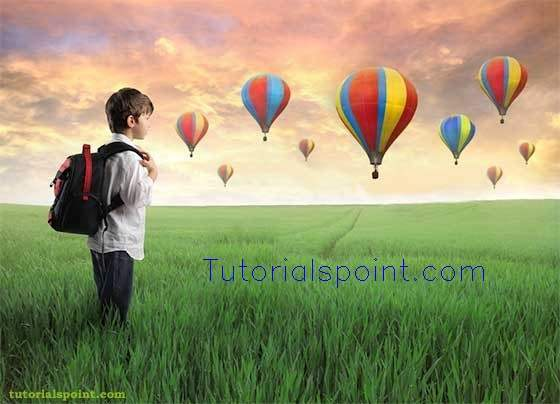## Applying Image Watermark on Image

We are going to use OpenCV function addWeighted to apply image watermark to image. It can be found under Core package. Its syntax is given below −

```Core.addWeighted(InputArray src1, alpha, src2 (Watermark image), beta, gamma, OutputArray dst);
```

The parameters of this function are described below −

Sr.No. Parameter & Description
1

src1

It is first input array.

2

alpha

It is the weight of the first array elements.

3

src2

It is the second input array of the same size and channel number as src1.

4

beta

It is the weight of the second array elements.

5

gamma

It is the scalar added to each sum.

6

dst

It is the output array that has the same size and number of channels as the input arrays.

### Example

The following example demonstrates the use of Core class to apply image watermark to an image −

```import org.opencv.core.Core;
import org.opencv.core.Mat;

import org.opencv.highgui.Highgui;
import org.opencv.imgproc.Imgproc;

public class Main {
public static void main( String[] args ) {

try{

Rect ROI = new Rect(waterMark.rows() * 4,waterMark.cols(),  waterMark.cols(),waterMark.rows());

Core.addWeighted(source.submat(ROI), 0.8, waterMark, 0.2, 1,  source.submat(ROI));
Highgui.imwrite("watermarkedImage.jpg", source);

} catch (Exception e) {
System.out.println("Error: " + e.getMessage());
}
}
}
```

### Output

When you execute the given code, the following output is seen −

#### Original Image#### The Watermark Image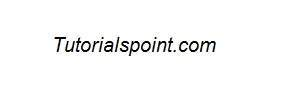#### Watermarked Image# Java DIP - Understand Convolution

Convolution is a mathematical operation on two functions f and g. The function f and g in this case are images, since an image is also a two dimensional function.

## Performing Convolution

In order to perform convolution on an image, following steps are taken −

• Flip the mask (horizontally and vertically) only once.
• Slide the mask onto the image.
• Multiply the corresponding elements and then add them.
• Repeat this procedure until all values of the image has been calculated.

We use OpenCV function filter2D to apply convolution to images. It can be found under Imgproc package. Its syntax is given below −

```filter2D(src, dst, depth , kernel, anchor, delta, BORDER_DEFAULT );
```

The function arguments are described below −

Sr.No. Argument & Description
1

src

It is source image.

2

dst

It is destination image.

3

depth

It is the depth of dst. A negative value (such as -1) indicates that the depth is the same as the source.

4

kernel

It is the kernel to be scanned through the image.

5

anchor

It is the position of the anchor relative to its kernel. The location Point (-1, -1) indicates the center by default.

6

delta

It is a value to be added to each pixel during the convolution. By default it is 0.

7

BORDER_DEFAULT

We let this value by default.

### Example

The following example demonstrates the use of Imgproc class to perform convolution on an image of Grayscale.

```import org.opencv.core.Core;
import org.opencv.core.CvType;
import org.opencv.core.Mat;

import org.opencv.highgui.Highgui;
import org.opencv.imgproc.Imgproc;

public class convolution {
public static void main( String[] args ) {

try {
int kernelSize = 3;

Mat destination = new Mat(source.rows(),source.cols(),source.type());

Mat kernel = new Mat(kernelSize,kernelSize, CvType.CV_32F) {
{
put(0,0,0);
put(0,1,0);
put(0,2,0);

put(1,0,0);
put(1,1,1);
put(1,2,0);

put(2,0,0);
put(2,1,0);
put(2,2,0);
}
};

Imgproc.filter2D(source, destination, -1, kernel);
Highgui.imwrite("original.jpg", destination);

} catch (Exception e) {
System.out.println("Error:" + e.getMessage());
}
}
}
```

### Output

In this example we convolve our image with the following filter(kernel). This filter results in producing original image as it is −

 0 0 0 0 1 0 0 0 0

#### Original Image#### Convolved Image# Java DIP - Prewitt Operator

Prewitt operator is used for edge detection in an image. It detects two types of edges: vertical edges and horizontal edges.

We use OpenCV function filter2D to apply Prewitt operator to images. It can be found under Imgproc package. Its syntax is given below −

```filter2D(src, dst, depth , kernel, anchor, delta, BORDER_DEFAULT );
```

The function arguments are described below −

Sr.No. Argument & Description
1

src

It is source image.

2

dst

It is destination image.

3

depth

It is the depth of dst. A negative value (such as -1) indicates that the depth is the same as the source.

4

kernel

It is the kernel to be scanned through the image.

5

anchor

It is the position of the anchor relative to its kernel. The location Point (-1, -1) indicates the center by default.

6

delta

It is a value to be added to each pixel during the convolution. By default it is 0.

7

BORDER_DEFAULT

We let this value by default.

Apart from the filter2D method, there are other methods provide by the Imgproc class. They are described briefly −

Sr.No. Method & Description
1

cvtColor(Mat src, Mat dst, int code, int dstCn)

It converts an image from one color space to another.

2

dilate(Mat src, Mat dst, Mat kernel)

It dilates an image by using a specific structuring element.

3

equalizeHist(Mat src, Mat dst)

It equalizes the histogram of a grayscale image.

4

filter2D(Mat src, Mat dst, int depth, Mat kernel, Point anchor, double delta)

It convolves an image with the kernel.

5

GaussianBlur(Mat src, Mat dst, Size ksize, double sigmaX)

It blurs an image using a Gaussian filter.

6

integral(Mat src, Mat sum)

It calculates the integral of an image.

## Example

The following example demonstrates the use of Imgproc class to apply Prewitt operator to an image of Grayscale.

```import org.opencv.core.Core;
import org.opencv.core.CvType;
import org.opencv.core.Mat;

import org.opencv.highgui.Highgui;
import org.opencv.imgproc.Imgproc;

public class convolution {
public static void main( String[] args ) {
try {
int kernelSize = 9;

Mat destination = new Mat(source.rows(),source.cols(),source.type());

Mat kernel = new Mat(kernelSize,kernelSize, CvType.CV_32F) {
{
put(0,0,-1);
put(0,1,0);
put(0,2,1);

put(1,0-1);
put(1,1,0);
put(1,2,1);

put(2,0,-1);
put(2,1,0);
put(2,2,1);
}
};

Imgproc.filter2D(source, destination, -1, kernel);
Highgui.imwrite("output.jpg", destination);

} catch (Exception e) {
System.out.println("Error: " + e.getMessage());
}
}
}
```

## Output

When you execute the given code, the following output is seen −

### Original ImageThis original image is convolved with the Prewitt operator of vertical edges as given below −

### Vertical direction

 -1 0 1 -1 0 1 -1 0 1

### Convolved Image(Vertical Direction)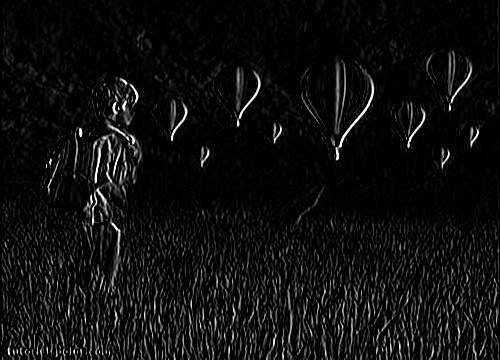This original image has also been convolved with the Prewitt operator of horizontal edges, which is given below −

### Horizontal Direction

 -1 -1 -1 0 0 0 1 1 1

### Convolved Image(Horizontal Direction)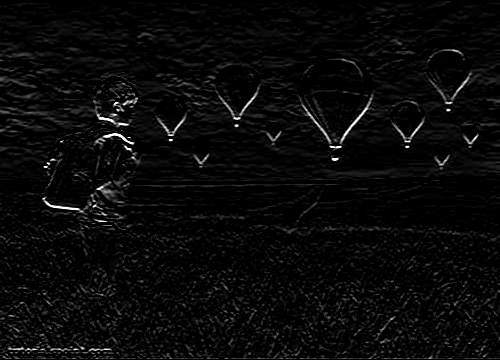# Java DIP - Sobel Operator

Sobel operator is very similar to Prewitt operator. It is also a derivative mask and is used for edge detection. Sobel operator is used to detect two kinds of edges in an image: Vertical direction edges and Horizontal direction edges.

We are going to use OpenCV function filter2D to apply Sobel operator to images. It can be found under Imgproc package. Its syntax is given below −

```filter2D(src, dst, depth , kernel, anchor, delta, BORDER_DEFAULT );
```

The function arguments are described below −

Sr.No. Argument
1

src

It is source image.

2

dst

It is destination image.

3

depth

It is the depth of dst. A negative value (such as -1) indicates that the depth is the same as the source.

4

kernel

It is the kernel to be scanned through the image.

5

anchor

It is the position of the anchor relative to its kernel. The location Point (-1, -1) indicates the center by default.

6

delta

It is a value to be added to each pixel during the convolution. By default it is 0.

7

BORDER_DEFAULT

We let this value by default.

Apart from the filter2D method, there are other methods provide by the Imgproc class. They are described briefly −

Sr.No. Method & Description
1

cvtColor(Mat src, Mat dst, int code, int dstCn)

It converts an image from one color space to another.

2

dilate(Mat src, Mat dst, Mat kernel)

It dilates an image by using a specific structuring element.

3

equalizeHist(Mat src, Mat dst)

It equalizes the histogram of a grayscale image.

4

filter2D(Mat src, Mat dst, int depth, Mat kernel, Point anchor, double delta)

It convolves an image with the kernel.

5

GaussianBlur(Mat src, Mat dst, Size ksize, double sigmaX)

It blurs an image using a Gaussian filter.

6

integral(Mat src, Mat sum)

It calculates the integral of an image.

## Example

The following example demonstrates the use of Imgproc class to apply Sobel operator to an image of Grayscale.

```import org.opencv.core.Core;
import org.opencv.core.CvType;
import org.opencv.core.Mat;

import org.opencv.highgui.Highgui;
import org.opencv.imgproc.Imgproc;

public class convolution {
public static void main( String[] args ) {

try {
int kernelSize = 9;

Mat destination = new Mat(source.rows(),source.cols(),source.type());

Mat kernel = new Mat(kernelSize,kernelSize, CvType.CV_32F) {
{
put(0,0,-1);
put(0,1,0);
put(0,2,1);

put(1,0-2);
put(1,1,0);
put(1,2,2);

put(2,0,-1);
put(2,1,0);
put(2,2,1);
}
};

Imgproc.filter2D(source, destination, -1, kernel);
Highgui.imwrite("output.jpg", destination);

} catch (Exception e) {
System.out.println("Error: " + e.getMessage());
}
}
}
```

## Output

When you execute the given code, the following output is seen −

### Original ImageThis original image is convolved with the Sobel operator of vertical edges, which is given below −

### Vertical Direction

 -1 0 1 -2 0 2 -1 0 1

### Convolved Image(Vertical Direction)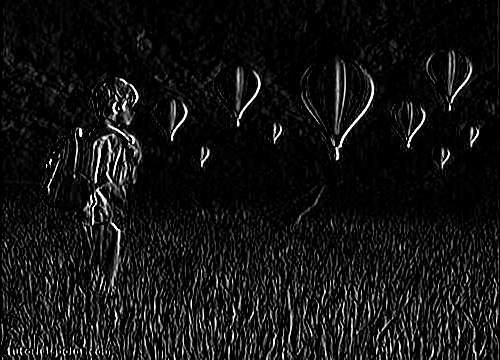This original is convolved with the Sobel operator of horizontal edges, which is given below −

### Horizontal Direction

 -1 -2 -1 0 0 0 1 2 1

### Convolved Image(Horizontal Direction)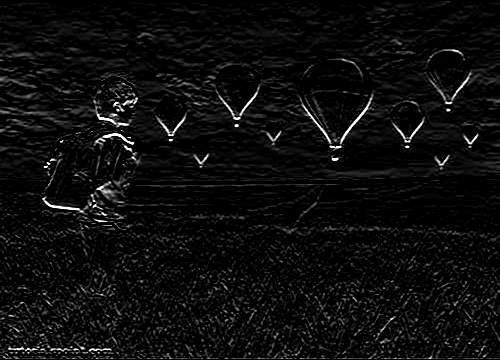# Java DIP - Kirsch Operator

Kirsch compass masks are yet another type of derivative mask which are used for edge detection. This operator is also known as direction mask. In this operator we take one mask and rotate it in all the eight compass directions to get edges of the eight directions.

We are going to use OpenCV function filter2D to apply Kirsch operator to images. It can be found under Imgproc package. Its syntax is given below −

```filter2D(src, dst, depth , kernel, anchor, delta, BORDER_DEFAULT );
```

The function arguments are described below −

Sr.No. Argument
1

src

It is source image.

2

dst

It is destination image.

3

depth

It is the depth of dst. A negative value (such as -1) indicates that the depth is the same as the source.

4

kernel

It is the kernel to be scanned through the image.

5

anchor

It is the position of the anchor relative to its kernel. The location Point (-1, -1) indicates the center by default.

6

delta

It is a value to be added to each pixel during the convolution. By default it is 0.

7

BORDER_DEFAULT

We let this value by default.

Apart from the filter2D() method, there are other methods provided by the Imgproc class. They are described briefly −

Sr.No. Method & Description
1

cvtColor(Mat src, Mat dst, int code, int dstCn)

It converts an image from one color space to another.

2

dilate(Mat src, Mat dst, Mat kernel)

It dilates an image by using a specific structuring element.

3

equalizeHist(Mat src, Mat dst)

It equalizes the histogram of a grayscale image.

4

filter2D(Mat src, Mat dst, int depth, Mat kernel, Point anchor, double delta)

It convolves an image with the kernel.

5

GaussianBlur(Mat src, Mat dst, Size ksize, double sigmaX)

It blurs an image using a Gaussian filter.

6

integral(Mat src, Mat sum)

It calculates the integral of an image.

## Example

The following example demonstrates the use of Imgproc class to apply Kirsch operator to an image of Grayscale.

```import org.opencv.core.Core;
import org.opencv.core.CvType;
import org.opencv.core.Mat;

import org.opencv.highgui.Highgui;
import org.opencv.imgproc.Imgproc;

public class convolution {
public static void main( String[] args ) {

try {
int kernelSize = 9;

Mat destination = new Mat(source.rows(),source.cols(),source.type());

Mat kernel = new Mat(kernelSize,kernelSize, CvType.CV_32F) {
{
put(0,0,-3);
put(0,1,-3);
put(0,2,-3);

put(1,0-3);
put(1,1,0);
put(1,2,-3);

put(2,0,5);
put(2,1,5);
put(2,2,5);
}
};

Imgproc.filter2D(source, destination, -1, kernel);
Highgui.imwrite("output.jpg", destination);

} catch (Exception e) {
System.out.println("Error: " + e.getMessage());
}
}
}
```

## Output

When you execute the given code, the following output is seen −

### Original ImageThis original image is convolved with the Kirsch operator of East edges, which as given below −

### Kirsch East

 -3 -3 -3 -3 0 -3 5 5 5

### Convolved Image(Kirsch East)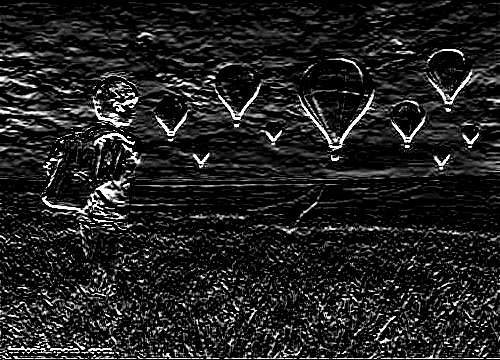This original image is convolved with the Kirsch operator of South West edges, which as given below −

### Kirsch South West

 5 5 -3 5 0 -3 -3 -3 -3

### Convolved Image (Kirsch South West)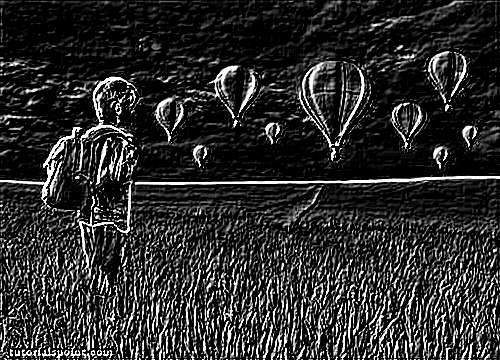# Java DIP - Robinson Operator

Robinson compass masks are yet another type of derivative masks which are used for edge detection. This operator is also known as direction mask. In this operator we take one mask and rotate it in all the eight major directions to get edges of the eight directions.

We are going to use OpenCV function filter2D to apply Robinson operator to images. It can be found under Imgproc package. Its syntax is given below −

```filter2D(src, dst, depth , kernel, anchor, delta, BORDER_DEFAULT );
```

The function arguments are described below −

Sr.No. Argument & Description
1

src

It is source image.

2

dst

It is destination image.

3

depth

It is the depth of dst. A negative value (such as -1) indicates that the depth is the same as the source.

4

kernel

It is the kernel to be scanned through the image.

5

anchor

It is the position of the anchor relative to its kernel. The location Point(-1, -1) indicates the center by default.

6

delta

It is a value to be added to each pixel during the convolution. By default it is 0.

7

BORDER_DEFAULT

We let this value by default.

Apart from the filter2D method, there are other methods provided by the Imgproc class. They are described briefly −

Sr.No. Method & Description
1

cvtColor(Mat src, Mat dst, int code, int dstCn)

It converts an image from one color space to another.

2

dilate(Mat src, Mat dst, Mat kernel)

It dilates an image by using a specific structuring element.

3

equalizeHist(Mat src, Mat dst)

It equalizes the histogram of a grayscale image.

4

filter2D(Mat src, Mat dst, int depth, Mat kernel, Point anchor, double delta)

It convolves an image with the kernel.

5

GaussianBlur(Mat src, Mat dst, Size ksize, double sigmaX)

It blurs an image using a Gaussian filter.

6

integral(Mat src, Mat sum)

It calculates the integral of an image.

## Example

The following example demonstrates the use of Imgproc class to apply Robinson operator to an image of Grayscale.

```import org.opencv.core.Core;
import org.opencv.core.CvType;
import org.opencv.core.Mat;

import org.opencv.highgui.Highgui;
import org.opencv.imgproc.Imgproc;

public class convolution {
public static void main( String[] args ) {

try {
int kernelSize = 9;

Mat destination = new Mat(source.rows(),source.cols(),source.type());

Mat kernel = new Mat(kernelSize,kernelSize, CvType.CV_32F) {
{
put(0,0,-1);
put(0,1,0);
put(0,2,1);

put(1,0-2);
put(1,1,0);
put(1,2,2);

put(2,0,-1);
put(2,1,0);
put(2,2,1);
}
};

Imgproc.filter2D(source, destination, -1, kernel);
Highgui.imwrite("output.jpg", destination);

} catch (Exception e) {
System.out.println("Error: " + e.getMessage());
}
}
}
```

## Output

When you execute the given code, the following output is seen −

### Original ImageThis original image is convolved with the Robinson operator of North edges as given below −

 -1 0 1 -2 0 2 -1 0 1

### Convolved Image(Robinson North)This original image has also been convolved with the Robinson operator of East edges as given below −

 -1 -2 -1 0 0 0 1 2 1

### Convolved Image(Robinson East)# Java DIP - Laplacian Operator

Laplacian Operator is also a derivative operator which is used to find edges in an image. The major difference between Laplacian and other operators like Prewitt, Sobel, Robinson, and Kirsch is that these all are first order derivative masks but Laplacian is a second order derivative mask.

We use OpenCV function filter2D to apply Laplacian operator to images. It can be found under Imgproc package. Its syntax is given below −

```filter2D(src, dst, depth , kernel, anchor, delta, BORDER_DEFAULT );
```

The function arguments are described below −

Sr.No. Arguments
1

src

It is source image.

2

dst

It is destination image.

3

depth

It is the depth of dst. A negative value (such as -1) indicates that the depth is the same as the source.

4

kernel

It is the kernel to be scanned through the image.

5

anchor

It is the position of the anchor relative to its kernel. The location Point (-1, -1) indicates the center by default.

6

delta

It is a value to be added to each pixel during the convolution. By default it is 0.

7

BORDER_DEFAULT

We let this value by default.

Apart from the filter2D() method, there are other methods provided by the Imgproc class. They are described briefly −

Sr.No. Method & Description
1

cvtColor(Mat src, Mat dst, int code, int dstCn)

It converts an image from one color space to another.

2

dilate(Mat src, Mat dst, Mat kernel)

It dilates an image by using a specific structuring element.

3

equalizeHist(Mat src, Mat dst)

It equalizes the histogram of a grayscale image.

4

filter2D(Mat src, Mat dst, int depth, Mat kernel, Point anchor, double delta)

It convolves an image with the kernel.

5

GaussianBlur(Mat src, Mat dst, Size ksize, double sigmaX)

It blurs an image using a Gaussian filter.

6

integral(Mat src, Mat sum)

It calculates the integral of an image.

## Example

The following example demonstrates the use of Imgproc class to apply Laplacian operator to an image of Grayscale.

```import org.opencv.core.Core;
import org.opencv.core.CvType;
import org.opencv.core.Mat;

import org.opencv.highgui.Highgui;
import org.opencv.imgproc.Imgproc;

public class convolution {
public static void main( String[] args ) {

try {
int kernelSize = 9;

Mat destination = new Mat(source.rows(),source.cols(),source.type());

Mat kernel = new Mat(kernelSize,kernelSize, CvType.CV_32F) {
{
put(0,0,0);
put(0,1,-1)
put(0,2,0);

put(1,0-1);
put(1,1,4);
put(1,2,-1);

put(2,0,0);
put(2,1,-1);
put(2,2,0);
}
};

Imgproc.filter2D(source, destination, -1, kernel);
Highgui.imwrite("output.jpg", destination);

} catch (Exception e) {
System.out.println("Error: " + e.getMessage());
}
}
}
```

## Output

When you execute the given code, the following output is seen −

### Original ImageThis original image is convolved with the Laplacian Negative operator as given below −

### Laplacian Negative

 0 -1 0 -1 4 -1 0 -1 0

### Convolved Image(Laplacian Negative)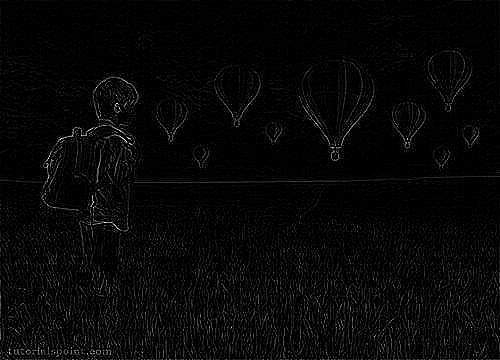This original image is convolved with the Laplacian Positive operator as given below −

### Laplacian Positive

 0 1 0 1 -4 1 0 1 0

### Convolved Image (Laplacian Positive)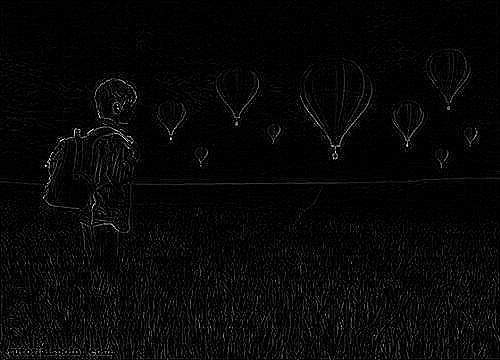# Java DIP - Weighted Average Filter

In weighted average filter, we gave more weight to the center value, due to which the contribution of center becomes more than the rest of the values. Due to weighted average filtering, we can control the blurring of image.

We use OpenCV function filter2D to apply weighted average filter to images. It can be found under Imgproc package. Its syntax is given below −

```filter2D(src, dst, depth , kernel, anchor, delta, BORDER_DEFAULT );
```

The function arguments are described below −

Sr.No. Argument & Description
1

src

It is source image.

2

dst

It is destination image.

3

ddepth

It is the depth of dst. A negative value (such as -1) indicates that the depth is the same as the source.

4

kernel

It is the kernel to be scanned through the image.

5

anchor

It is the position of the anchor relative to its kernel. The location Point(-1, -1) indicates the center by default.

6

delta

It is a value to be added to each pixel during the convolution. By default it is 0.

7

BORDER_DEFAULT

We let this value by default.

Apart from the filter2D() method, there are other methods provide by the Imgproc class. They are described briefly −

Sr.No. Method & Description
1

cvtColor(Mat src, Mat dst, int code, int dstCn)

It converts an image from one color space to another.

2

dilate(Mat src, Mat dst, Mat kernel)

It dilates an image by using a specific structuring element.

3

equalizeHist(Mat src, Mat dst)

It equalizes the histogram of a grayscale image.

4

filter2D(Mat src, Mat dst, int depth, Mat kernel, Point anchor, double delta)

It convolves an image with the kernel.

5

GaussianBlur(Mat src, Mat dst, Size ksize, double sigmaX)

It blurs an image using a Gaussian filter.

6

integral(Mat src, Mat sum)

It calculates the integral of an image.

## Example

The following example demonstrates the use of Imgproc class to apply weighted average filter to an image of Graycale.

```import org.opencv.core.Core;
import org.opencv.core.CvType;
import org.opencv.core.Mat;

import org.opencv.highgui.Highgui;
import org.opencv.imgproc.Imgproc;

public class convolution {
public static void main( String[] args ) {

try {
int kernelSize = 9;

Mat destination = new Mat(source.rows(),source.cols(),source.type());

Mat kernel = Mat.ones(kernelSize,kernelSize, CvType.CV_32F) {

for(int i=0; i<kernel.rows(); i++) {
for(int j=0; j<kernel.cols(); j++) {

double[] m = kernel.get(i, j);

for(int k =0; k<m.length; k++) {

if(i==1 && j==1) {
m[k] = 10/18;
}
else{
m[k] = m[k]/(18);
}
}
kernel.put(i,j, m);

}
}
};

Imgproc.filter2D(source, destination, -1, kernel);
Highgui.imwrite("output.jpg", destination);

} catch (Exception e) {
System.out.println("Error: " + e.getMessage());
}
}
}
```

## Output

When you execute the given code, the following output is seen −

### Original ImageThis original image is convolved with the weighted average filter as given below −

### Weighted Average Filter

 1 1 1 1 10 1 1 1 1

### Convolved Image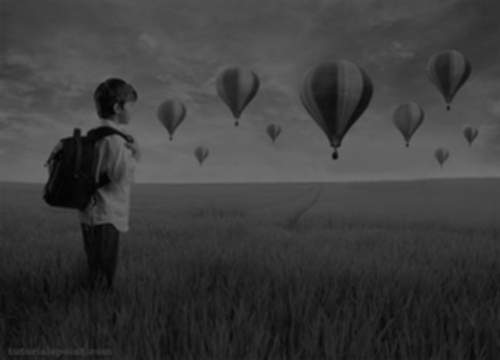# Java DIP - Create Zooming Effect

Zooming is the process of enlarging an image so that the details in the image become more visible and prominent.

We use OpenCV function resize to apply zooming to images. It can be found under Imgproc package. Its syntax is given below −

```Imgproc.resize(source,destination, destination.size(),zoomFactor,zoomFactor,Interpolation);
```

In the resize function, we pass source image, destination image and its size, zooming factor, and the interpolation method to use.

The interpolation methods available are described below −

Sr.No. Interpolation method & Description
1

INTER_NEAREST

It is nearest-neighbour interpolation.

2

INTER_LINEAR

It is bilinear interpolation (used by default).

3

INTER_AREA

It is resampling using pixel area relation. It may be a preferred method for image decimation, as it gives more-free results.

4

INTER_CUBIC

It is a bi-cubic interpolation over 4x4 pixel neighbourhood.

5

INTER_LANCZOS4

It is a Lanczos interpolation over 8x8 pixel neighbourhood.

Apart from the resize method, there are other methods provided by the Imgproc class. They are described briefly −

Sr.No. Method & Description
1

cvtColor(Mat src, Mat dst, int code, int dstCn)

It converts an image from one color space to another.

2

dilate(Mat src, Mat dst, Mat kernel)

It dilates an image by using a specific structuring element.

3

equalizeHist(Mat src, Mat dst)

It equalizes the histogram of a grayscale image.

4

filter2D(Mat src, Mat dst, int ddepth, Mat kernel, Point anchor, double delta)

It convolves an image with the kernel.

5

GaussianBlur(Mat src, Mat dst, Size ksize, double sigmaX)

It blurs an image using a Gaussian filter.

6

integral(Mat src, Mat sum)

It calculates the integral of an image.

## Example

The following example demonstrates the use of Imgproc class to apply zooming to an image.

```import org.opencv.core.Core;
import org.opencv.core.Mat;

import org.opencv.highgui.Highgui;
import org.opencv.imgproc.Imgproc;

public class Main {
public static void main( String[] args ) {

try {
int zoomingFactor = 2;

Mat destination = new Mat(source.rows() * zoomingFactor, source.cols()*  zoomingFactor,source.type());

Imgproc.resize(source, destination, destination.size(),  zoomingFactor,zoomingFactor,Imgproc.INTER_NEAREST);
Highgui.imwrite("zoomed.jpg", destination);

} catch (Exception e) {
System.out.println("Error: "+e.getMessage());
}
}
}
```

## Output

When you execute the given code, the following output is seen −

### Original Image### Zoomed Image(Zooming factor − 2)# Java DIP - Open Source Libraries

In this chapter, we explore some of the free image processing libraries that are widely used and can be easily integrated in the project. These libraries include −

• ImageJ
• Fiji
• Commons Imaging
• ImageMagick
• Endrov
• OpenCv

## ImageJ

ImageJ is a public domain Java image processing program inspired by NIH Image for the Macintosh. It can display, edit, analyze, process, save, and print 8-bit, 16-bit, and 32-bit images.

Some of the basic features of ImageJ are described below −

Sr.No. Feature & Description
1

Runs Everywhere

ImageJ is written in Java, which allows it to run on Linux, Mac OS X and Windows, in both 32-bit and 64-bit modes.

2

Open Source

ImageJ and its Java source code are freely available and in the public domain.

3

Toolkit

Use ImageJ as an image processing toolkit (class library) to develop applets, servlets, or applications.

4

Data Types

8-bit grayscale or indexed color, 16-bit unsigned integer, 32-bit floating-point, and RGB color.

5

File Formats

Open and save GIF, JPEG, BMP, PNG, PGM, FITS, and ASCII. Open DICOM. Open TIFFs, GIFs, JPEGs, DICOMs, and raw data using a URL.

6

Selections

Create rectangular, elliptical, or irregular area selections. Create line and point selections.

7

Image Enhancement

Supports smoothing, sharpening, edge detection, median filtering, and thresholding on both 8-bit grayscale and RGB color images.

8

Color Processing

Split a 32-bit color image into RGB or HSV components. Merge 8-bit components into a color image.

## Fiji

Fiji is an image processing package. It can be described as a distribution of ImageJ (and ImageJ2) together with Java, Java3D, and a lot of plug-ins organized into a coherent menu structure. Fiji compares to ImageJ as Ubuntu compares to Linux.

Apart from the ImageJ basic features, some of the advanced features of Fiji are described below −

Sr.No. Feature & Description
1

Registering 3D images

This involves Elastic Alignment and Montage, Feature Extraction, Image Stabilizer etc.

2

Segmenting images

It offers more than 35 types of segmentation.

3

Useful keyboard short cuts

Fuji has a lot of keyboard short-cuts.

4

Scripting

Allow scripting with Macros, in JavaScript, JRuby, Jython, Clojure, and Beanshell.

5

Developing Plug-ins

Use the Script Editor to start developing plug-ins and then run the plug-ins.

6

ImageJ Tricks

ImageJ is easy to use, but sometimes you wish for some function that is actually implemented, yet you do not know how to trigger.

## Commons Imaging

Apache Commons Imaging, previously known as Apache Commons Sanselan, is a library that reads and writes a variety of image formats, including fast parsing of image information such as(size, color, space, ICC profile, etc.) and the meta data.

Some of the basic features of ImageJ are described below −

Sr.No. Feature & Description
1

Java

Apache Commons Imaging is written in 100% pure Java. It executes on any JVM, and any platform, without modification.

2

Image Formats

It reads and writes a wide variety of image formats, and supports some variations and encodings missed by all or most other libraries.

3

It supports reading and writing a variety of meta data in a structured way, including EXIF meta data.

4

Network Friendly

It is network-friendly. Commons Imaging only reads the data it needs, and caches what is read so that it is not too heavy on the network.

5

Easy to use

It is designed to be very easy to use. It has a simple, clean interface. Most operations are a single Imaging method calls.

6

Transparent

Commons Imaging aims to be transparent. There are no hidden buffers to dispose, no native memory to free, no background threads.

7

Open Source

It is Free Software/Open Source. It is available under the Apache Software License.

8

Color Conversions

The ColorConversions class offers methods to convert between the following color spaces − CIE-L*CH, CIE-L*ab, CIE-L*uv, CMY, CMYK, HSL, HSV, Hunter-Lab, RGB, XYZ, and YXY.

## ImageMagick

ImageMagick is a software suite to create, edit, compose, or convert bitmap images. It can read and write images in more than 100 formats including DPX, EXR, GIF, JPEG, JPEG-2000, PDF, PNG, Postscript, SVG, and TIFF. Use ImageMagick to resize, flip, mirror, rotate, distort, shear, and transform images, adjust image colors, apply various special effects, or draw text, lines, polygons, ellipses, and Bezier curve.

Some of the basic features of ImageMagick are described below −

Sr.No. Feature & Description
1

Format conversion

It converts an image from one format to another (e.g. PNG to JPEG).

2

Transform

It can resize, rotate, crop, flip or trim an image.

3

Transparency

It renders portions of an image invisible.

4

Draw

It adds shapes or text to an image.

5

Decorate

It adds a border or frame to an image.

6

Special effects

It can Blur, sharpen, threshold, or tint an image.

7

Animation

It can create a GIF animation sequence from a group of images.

8

Composite

It can overlap one image over another.

9

Morphology of shapes

It extracts features, describe shapes and recognize patterns in images.

10

Encipher or decipher an image

It converts ordinary images into unintelligible gibberish and back again.

## Endrov

Endrov is a multi-purpose image analysis program. It is written independently and designed to address many of the shortcomings of other free software and many commercial packages.

Some of the basic features of Endrov are described below −

Sr.No. Feature & Description
1

View data

It views data, in 2D and 3D. Designed to handle complex 4D data schemes and unlimited number of channels, where each channel can have its own X, Y, and Z resolution.

2

It annotates your images, automatically or by hand, to understand them and get statistics.

3

Undo and Redo

It can undo and redo for all operations.

4

Lazy Evaluation

It is designed from the ground to handle large image sets. Endrov uses lazy evaluation, a concept mostly available in research programming languages.

5

Scripting language

It supports graphical scripting language, as well as traditional scripting.

6

Java

Written in Java. Plug-in architecture allows easy extension with new Java plug-ins. It can interact with Matlab.

7

Formats

It accesses almost all commercial and open file formats using Bio-formats.

8

Microscopic Processing

It can control all your microscopes with one program and do on-the-fly image analysis.

LEADTOOLS provides over 200 image processing functions in several categories including document cleanup, medical image enhancement, color conversion and correction, noise reduction, edge detection, and more.

Some of the basic features of LEADTOOLS are described below −

Sr.No. Feature & Description
1

Scanned Document Image Processing

This powerful collection of functions can read scanned documents of artefacts and imperfections such as punched holes, skewed angles, borders, dust speckles, and more.

2

Medical Image Processing

Enhance the image or highlight the details by shifting, selecting, subtracting, and removing the background for better visuals.

3

Geometric Transformation

These functions can be used to clean, align, correct images, or apply artistic 3D effects.

4

Brightness and Contrast

These functions can be used to enhance images, apply artistic effects, or aid in diagnostic evaluation of medical images.

5

Color Space Conversion

They can add image color space functionality to single and multi-threaded applications including IIS and Windows WF hosted applications.

6

Color Correction

These functions are used to correct images with swapped color channels, balance color intensities or perform various image analysis tasks.

7

Image Enhancement

These functions are used to correct common errors in photography such as red-eye and imbalanced colors as well as aid in diagnostic evaluation of medical images.

8

Region of Interest

These functions are used to create and modify regions of interest in images to perform image processing functions on specific portions of an image, save time in bar-code, and OCR recognition or perform various image analysis tasks.

## OpenCV

OpenCV is released under a BSD license and hence it is free for both academic and commercial use. It has C++, C, Python, and Java interfaces and it supports Windows, Linux, Mac OS, iOS, and Android. OpenCV was designed for computational efficiency and with a strong focus on real-time applications. Written in optimized C/C++, the library can take advantage of multi-core processing.

Some basic features of OpenCV are described briefly −

Sr.No. Feature & Description
1

Smoothing Images

This involves applying Blur, GaussianBlur, medianBlur and bilateral Filter.

2

Eroding and Dilating

It can apply two very common morphology operators − Dilation and Erosion.

3

Morphology Transformations

OpenCV function morphologyEx to apply Morphological Transformation such as opening, closing, TopHat, and BlackHat etc.

4

Image Pyramids

OpenCV functions pyrUp and pyrDown to down sample or up sample a given image.

4

Basic Thresholding Operations

Perform basic thresholding operations using OpenCV function threshold.

5

OpenCV function copyMakeBorder is used to set the borders (extra padding to your image).

7

Remapping

In OpenCV, the function remap offers a simple remapping implementation.

8

Histogram Calculation

For simple purposes, OpenCV implements the function calcHist, which calculates the histogram of a set of arrays (usually images or image planes). It can operate with up to 32 dimensions.

# Java DIP - Introduction to OpenCV

OpenCV is released under a BSD license and hence it is free for both academic and commercial use. It has C++, C, Python, and Java interfaces, and it supports Windows, Linux, Mac OS, iOS, and Android.

OpenCV was designed for computational efficiency and with a strong focus on real-time applications. Written in optimized C/C++, the library can take advantage of multi-core processing.

Some of the basic features of OpenCV are described below −

Sr.No. Feature & Description
1

Smoothing Images

This involves applying Blur, GaussianBlur, medianBlur, and bilateral Filter.

2

Eroding and Dilating

It can apply two very common morphology operators − Dilation and Erosion.

3

Morphology Transformations

OpenCV function morphologyEx to apply Morphological Transformation such as opening, closing, TopHat, and BlackHat etc.

4

Image Pyramids

OpenCV functions pyrUp and pyrDown to down sample or up sample a given image.

4

Basic Thresholding Operations

It can perform basic thresholding operations using OpenCV function threshold.

5

OpenCV function copyMakeBorder is used to set the borders(extra padding to your image).

7

Remapping

In OpenCV, the function remap offers a simple remapping implementation.

8

Histogram Calculation

For simple purposes, OpenCV implements the function calcHist, which calculates the histogram of a set of arrays (usually images or image planes). It can operate with up to 32 dimensions.

## Integrating OpenCV

These following steps explain how to integrate OpenCV into your applications.

### Create User Library

Further, we create a user library of OpenCV, so that we can use it as a future project.

Launch Eclipse

Select Window -> Preferences from the menu.

Navigate under Java -> Build Path -> User Libraries and click New.Now enter the name for your library. For example, OpenCV-2.4.6.

After that, select your new user library(i.e. OpenCV-2.4.6) and click on Add External JARs.

Browse through C:\OpenCV-2.4.6\build\java\ and select opencv-246.jar. After adding the jar, extend the opencv-246.jar and select Native library location and press Edit.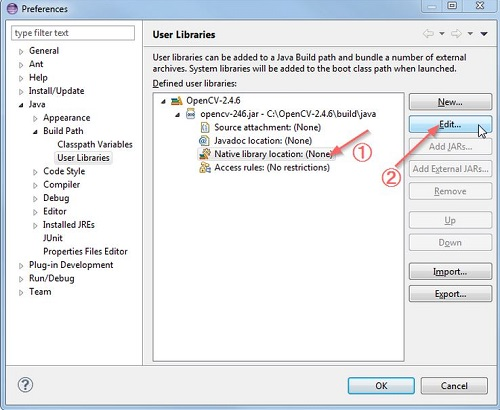Select External Folder... and browse to select the folder C:\OpenCV-2.4.6\build\java\x64. If you have a 32-bit system, you need to select the x86 folder instead of x64.

Press Ok and you are done.

Now your user library is created. Now you can reuse this configuration in any of the project.

### Create OpenCV Project

Create a new java project in eclipse.

On the Java Settings step, under Libraries tab, select Add Library... and select OpenCV-2.4.6, then click Finish.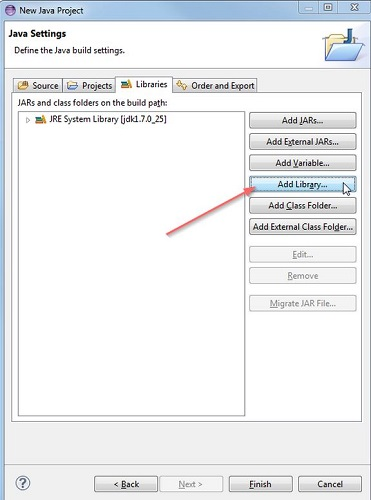Click finish and you are done.

# Java DIP - OpenCV GrayScale Conversion

In order to convert a color image to Grayscale image using OpenCV, we read the image into BufferedImage and convert it into Mat Object. Its syntax is given below −

```File input = new File("digital_image_processing.jpg");
//convert Buffered Image to Mat.
```

Then you can transform the image from RGB to Grayscale format by using method cvtColor() in the Imgproc class. Its syntax is given below −

```Imgproc.cvtColor(source mat, destination mat1, Imgproc.COLOR_RGB2GRAY);
```

The method cvtColor() takes three parameters which are the source image matrix, the destination image matrix, and the color conversion type.

Apart from the cvtColor method, there are other methods provided by the Imgproc class. They are listed below −

Sr.No. Method & Description
1

cvtColor(Mat src, Mat dst, int code, int dstCn)

It converts an image from one color space to another.

2

dilate(Mat src, Mat dst, Mat kernel)

It dilates an image by using a specific structuring element.

3

equalizeHist(Mat src, Mat dst)

It equalizes the histogram of a grayscale image.

4

filter2D(Mat src, Mat dst, int depth, Mat kernel, Point anchor, double delta)

It convolves an image with the kernel.

5

GaussianBlur(Mat src, Mat dst, Size ksize, double sigmaX)

It blurs an image using a Gaussian filter.

6

integral(Mat src, Mat sum)

It calculates the integral of an image.

## Example

The following example demonstrates the use of Imgproc class to convert an image to Grayscale −

```import java.awt.image.BufferedImage;
import java.awt.image.DataBufferByte;

import java.io.File;
import javax.imageio.ImageIO;

import org.opencv.core.Core;
import org.opencv.core.CvType;
import org.opencv.core.Mat;
import org.opencv.imgproc.Imgproc;

public class Main {
public static void main( String[] args ) {

try {
File input = new File("digital_image_processing.jpg");

byte[] data = ((DataBufferByte) image.getRaster().getDataBuffer()).getData();
Mat mat = new Mat(image.getHeight(), image.getWidth(), CvType.CV_8UC3);
mat.put(0, 0, data);

Mat mat1 = new Mat(image.getHeight(),image.getWidth(),CvType.CV_8UC1);
Imgproc.cvtColor(mat, mat1, Imgproc.COLOR_RGB2GRAY);

byte[] data1 = new byte[mat1.rows() * mat1.cols() * (int)(mat1.elemSize())];
mat1.get(0, 0, data1);
BufferedImage image1 = new BufferedImage(mat1.cols(),mat1.rows(), BufferedImage.TYPE_BYTE_GRAY);
image1.getRaster().setDataElements(0, 0, mat1.cols(), mat1.rows(), data1);

File ouptut = new File("grayscale.jpg");
ImageIO.write(image1, "jpg", ouptut);

} catch (Exception e) {
System.out.println("Error: " + e.getMessage());
}
}
}
```

## Output

When you execute the given example, it converts an image name digital_image_processing.jpg to its equivalent Grayscale image and writes it on hard disk with name grayscale.jpg.

### Original Image### Grayscale Image# Java DIP - OpenCV Color Space Conversion

In order to change color space of one image to another using OpenCV, we read image into BufferedImage and convert it into Mat Object. Its syntax is given below −

```File input = new File("digital_image_processing.jpg");
//convert Buffered Image to Mat.
```

OpenCv allows many color conversion types, all of which can be found in the Imgproc class. Some of the types are described briefly −

Sr.No. Color Conversion Type
1 COLOR_RGB2BGR
2 COLOR_RGB2BGRA
3 COLOR_RGB2GRAY
4 COLOR_RGB2HLS
5 COLOR_RGB2HSV
6 COLOR_RGB2Luv
7 COLOR_RGB2YUV
8 COLOR_RGB2Lab

From any of the color conversion type, just pass the appropriate one into method cvtColor() in the Imgproc class. Its syntax is given below −

```Imgproc.cvtColor(source mat, destination mat1, Color_Conversion_Code);
```

The method cvtColor() takes three parameters which are the source image matrix, the destination image matrix and the color conversion type.

Apart from the cvtColor() method, there are other methods provide by the Imgproc class. They are described briefly −

Sr.No. Method & Description
1

cvtColor(Mat src, Mat dst, int code, int dstCn)

It converts an image from one color space to another.

2

dilate(Mat src, Mat dst, Mat kernel)

It dilates an image by using a specific structuring element.

3

equalizeHist(Mat src, Mat dst)

It equalizes the histogram of a grayscale image.

4

filter2D(Mat src, Mat dst, int ddepth, Mat kernel, Point anchor, double delta)

It convolves an image with the kernel.

5

GaussianBlur(Mat src, Mat dst, Size ksize, double sigmaX)

It blurs an image using a Gaussian filter.

6

integral(Mat src, Mat sum)

It calculates the integral of an image.

## Example

The following example demonstrates the use of Imgproc class to convert an image from one color space to another.

```import java.awt.image.BufferedImage;
import java.awt.image.DataBufferByte;

import java.io.File;
import javax.imageio.ImageIO;

import org.opencv.core.Core;
import org.opencv.core.CvType;
import org.opencv.core.Mat;
import org.opencv.imgproc.Imgproc;

public class Main {
public static void main( String[] args ) {

try {
File input = new File("digital_image_processing.jpg");
byte[] data = ((DataBufferByte) image.getRaster().getDataBuffer()).getData();
Mat mat = new Mat(image.getHeight(),image.getWidth(), CvType.CV_8UC3);
mat.put(0, 0, data);

Mat mat1 = new Mat(image.getHeight(), image.getWidth(), CvType.CV_8UC3);
Imgproc.cvtColor(mat, mat1, Imgproc.COLOR_RGB2HSV);

byte[] data1 = new byte[mat1.rows()*mat1.cols()*(int)(mat1.elemSize())];
mat1.get(0, 0, data1);
BufferedImage image1 = new BufferedImage(mat1.cols(), mat1.rows(), 5);
image1.getRaster().setDataElements(0, 0, mat1.cols(), mat1.rows(), data1);

File ouptut = new File("hsv.jpg");
ImageIO.write(image1, "jpg", ouptut);

} catch (Exception e) {
System.out.println("Error: " + e.getMessage());
}
}
}
```

## Output

When you execute the given example, it converts an image name digital_image_processing.jpg to its equivalent HSV color space image and writes it on hard disk with name hsv.jpg.

### Original Image (RGB)### Converted Image (HSV)